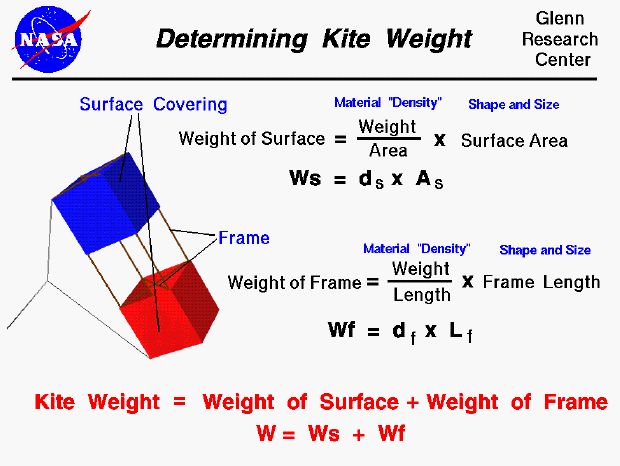A kite is able to fly because it generates a lift force that is greater than the weight of the kite. From Newton's first law of motion the net, vertical, external force on the kite accelerates it vertically and the kite flies. Kites depend on surfaces to generate the aerodynamic forces necessary for flight by deflecting the wind that blows past the surfaces. Most kites use a rigid frame to support the surfaces and transmit the forces. Different kites. have different arrangements of surfaces and frames, but we will use a simple box kite as an example on this page.

The flight performance of a kite depends on the relative size of the lift, drag, and weight forces that act on the kite. So, during design, we have to be able to determine the weight of the kite before we build it. Since the kite is composed of only surfaces and a frame, the weight of the kite, W, is equal to the weight of the surfaces, Ws, plus the weight of the frame, Wf.

W = Ws + Wf

The surfaces of the kite are made of a thin covering of paper, plastic, or cloth, which deflects the wind downward and creates the aerodynamic forces of lift and drag on the kite. The weight of the surface depends on the shape and size of the surface and on the material that is used to make the surface. We characterize the size and shape by the area of the surface. (You learn how to calculate areas based on size and shape in middle school). We characterize the surface material by a "density"; a weight per unit area of the material. We put the density in quotes because density is a material property normally defined to be the mass divided by the volume. Since we are dealing with very thin coverings which are nearly the same for all materials, we can use area instead of volume in the definition. And since all the materials are subjected to the same gravitational acceleration we can use weight instead of mass in our definition. The surface material density is determined by weighing a sample of the material on a scale, measuring the area of the sample, and performing the division. The weight of the surface is then the product of the surface material density ,ds, and the surface area, As.

Ws = ds * As

The kite frame is made from several sticks and some string. The sticks are made of a light, strong piece of wood such as balsa or bamboo, or a plastic tube. The weight of the frame depends on the shape and size of the frame and on the material used to make the frame. We characterize the size and shape by the total length of all of the sticks used in the frame. We characterize the frame material by a "density"; a weight per unit length of the material. Notice that this definition of "density" is different from the standard mass divided by volume and from the surface definition of weight divided by area. We use this definition because we are dealing with long thin sticks in the frame and the material is uniform along the length of the sticks. We determine the frame material density by weighing a sample on a scale, measuring the length of the sample, and performing the division. The weight of the frame is then the product of the frame material density, df, and the length of the frame, Lf.

Wf = df * Lf

Suppose we have a design that uses a 1/8 square inch balsa frame, and we change to a 1/4 square inch balsa frame. Even though we are using the same material (balsa), we have to change the frame material density in the weight calculation. The frame material density depends on the weight per length and the thicker 1/4 balsa has more weight per length than the 1/8.

You can use the KiteModeler computer program to see representative values for the surface and the frame material densities. Select "Design" mode and push the "Material" button on the design input panel. You can then use the drop menu for the surface and frame to display the density.